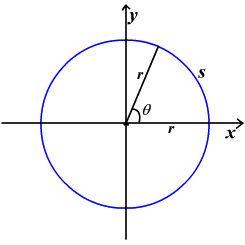# Radian Measure of an Angle

The measure of an angle is determined by the amount of rotation from the initial side to the terminal side.  One way to measure angles is in radians.  To define a radian , use a central angle of a circle (an angle whose vertex is the center of the circle).  One radian is the measure of a central angle that intercepts an arc $s$ equal in length to the radius $r$ of the circle.Since the circumference of a circle is $2\pi r$ , one revolution around a circle of radius $r$ corresponds to an angle of $2\pi$ radians because $\frac{s}{r}=\frac{2\pi r}{r}=2\pi \text{\hspace{0.17em}}\text{radians}.$